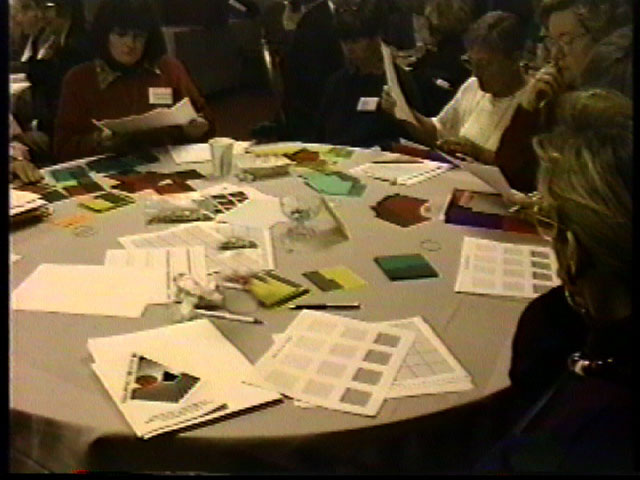Home
 REPRODUCIBLE MATERIALS .The seven files below contain grids tables and various types of Blank Decimal Squares. Workshop table with Blank Decimal Squares Decimal Squares Grid This grid is used for the activities in the steps for multiplication and division in the Decimal Squares Teacher's Guide. It is a 5 x 7 array of unit squares with each square divided into 10 x 10 smaller squares so that the linear dimensions of the sides of each unit square are in tenths of a unit and the area of each of the small squares within a unit square is one-hundredth of a square unit. Blank Decimal Squares for Hundredths Blank hundredths squares can be shaded to represent decimals and mixed decimals and to illustrate inequalities and the four basic operations on decimals. In particular they can be shaded to illustrate percents both less than and greater than 100% and to solve problems involving percents as outlined in the Decimal Squares Teacher's Guide. Blank Decimal Squares Mixed This page contains blank squares for tenths hundredths and thousandths which can be shaded to represent decimals and mixed decimals and to illustrate equalities inequalities and the four basic operations on decimals. Blank Decimal Squares for Tenths Blank tenths squares can be shaded to represent decimals and mixed decimals and to illustrate inequalities and the four basic operations on decimals. They can also be shaded to illustrate tenths of a percents and percents greater than 100%. Blank Decimal Squares for Thousandth Blank thousandth squares can be shaded to represent decimals and mixed decimals and to illustrate inequalities and the four basic operations on decimals. In particular they can be shaded to illustrate tenths of a percents such as 37.2% where each tenth of a percent is one of the small tiny thousandth squares. Decimal Squares Number Lines These number lines are divided into tenth and hundredths and can be used to represent mixed decimals in tenths and hundredths. They are also designed for the games and activities in the Decimal Squares Teacher's Guide for illustrating addition and subtraction of decimals and multiplication of a decimal by a whole number. Place Value Tables These tables are for representing three-place mixed decimals with two-place whole number parts. They are designed for the games and activities in the Decimal Squares Teacher's Guide that involve decimal place value addition and subtraction.